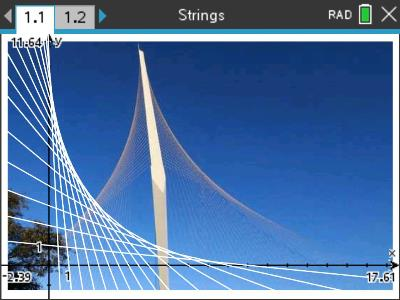# Activities

•• ##### Author60 Minutes

• ##### Device
•TI-Nspire™ CX CAS

4.5

## String Graphs Part1#### Activity Overview

This is part one of a four part series. In this activity students use the gradient and intercept form of a straight line to form a curved envelope similar to that found on the Chords bridge. In the second half of this activity students learn to use a parameter so that the family of graphs can be generated using a single equation.

#### Objectives

Students determine the equation to a straight line given the y intercept and information about the gradient. Students find patterns in the series of straight lines in order to generate a single equation for the family of straight lines. Specific calculator techniques are also covered in the activity.